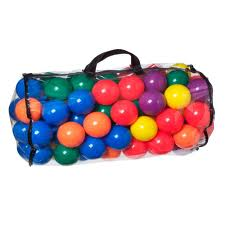# Bags, Balls and colours

Probability Level 2In a bag, there are some balls of the same size that are colored by $7$ colors, and for each color the number of balls is $77$. At least how many balls are needed to be picked out to ensure that one can obtain $7$ groups of $7$ balls each such that in each group the balls are monochromatic?

Note: Monochromatic means that all balls in the group are same in color. The balls in different groups can have the same color. For example, if we had 49 balls of the first color, then we are done.

×